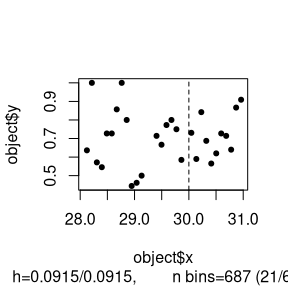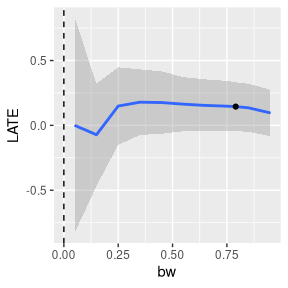# Morocco

#### 2020-08-05

we use the data from the Initiative Nationale du Development Humaine (INDH) a development project in Morocco. The data is included with the rddtools package under the name indh.

library(rddtools)
data("indh")

Now that we have loading the data we can briefly inspect the structure of the data

str(indh)
#> 'data.frame':    720 obs. of  2 variables:
#>  $choice_pg: int 0 1 1 1 1 1 0 1 0 0 ... #>$ poverty  : num  30.1 30.1 30.1 30.1 30.1 ...

The indh object is a data.frame containing 720 observations (representing individuals) of two variables:

• choice_pg
• poverty

The variable of interest is choice_pg, which represent the decision to contibute to a public good or not. The observations are individuals choosing to contribute or not, these individuals are clustered by the variable poverty which is the municiple structure at which funding was distributed as part of the INDH project. The forcing variable is poverty which represents the number of households in a commune living below the poverty threshold. As part of the INDH, commune with a proportion of household below the poverty threshhold greater than 30% were allowed to distribute the funding using a Community Driven Development scheme. The cutoff point for our analysis is therefore 30.

We can now transform the data.frame to a special rdd_data data.frame using the rdd_data() function.

rdd_dat_indh <- rdd_data(y=choice_pg,
x=poverty,
data=indh,
cutpoint=30 )

The structure is similar but contains some additional information.

str(rdd_dat_indh)
#> Classes 'rdd_data' and 'data.frame': 720 obs. of  2 variables:
#>  $x: num 30.1 30.1 30.1 30.1 30.1 ... #>$ y: int  0 1 1 1 1 1 0 1 0 0 ...
#>  - attr(*, "hasCovar")= logi FALSE
#>  - attr(*, "labels")= list()
#>  - attr(*, "cutpoint")= num 30
#>  - attr(*, "type")= chr "Sharp"

In order to best understand our data, we start with an exploratory data analysis using tables…

summary(rdd_dat_indh)
#> ### rdd_data object ###
#>
#> Cutpoint: 30
#> Type: Sharp
#> Sample size:
#>  -Full : 720
#>  -Left : 362
#>  -Right: 358
#> Covariates: no

…and plots.

plot(rdd_dat_indh[1:715,])We can now continue with a standard Regression Discontinuity Design (RDD) estimation.

(reg_para <- rdd_reg_lm(rdd_dat_indh, order=4))
#> ### RDD regression: parametric ###
#>  Polynomial order:  4
#>  Slopes:  separate
#>  Number of obs: 720 (left: 362, right: 358)
#>
#>  Coefficient:
#>   Estimate Std. Error t value Pr(>|t|)
#> D  0.22547    0.17696  1.2741    0.203

In addition to the parametric estimation, we can also perform a non-parametric estimation.

bw_ik <- rdd_bw_ik(rdd_dat_indh)
(reg_nonpara <- rdd_reg_np(rdd_object=rdd_dat_indh, bw=bw_ik))
#> ### RDD regression: nonparametric local linear###
#>  Bandwidth:  0.790526
#>  Number of obs: 460 (left: 139, right: 321)
#>
#>  Coefficient:
#>   Estimate Std. Error z value Pr(>|z|)
#> D 0.144775   0.095606  1.5143     0.13

Sensitity tests.

plotSensi(reg_nonpara, from=0.05, to=1, by=0.1)